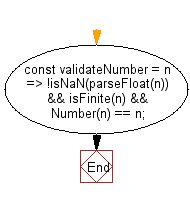# JavaScript: Return true if the given value is a number, false otherwise

## JavaScript fundamental (ES6 Syntax): Exercise-105 with Solution

Write a JavaScript program that return true if the given value is a number, false otherwise.

• Use parseFloat() to try to convert n to a number.
• Use !Number.isNaN() to check if num is a number.
• Use Number.isFinite() to check if num is finite.
• Use Number() and the loose equality operator (==) to check if the coercion holds.

Sample Solution:

JavaScript Code:

``````//#Source https://bit.ly/2neWfJ2
const validateNumber = n => !isNaN(parseFloat(n)) && isFinite(n) && Number(n) == n;
console.log(validateNumber('200'));
console.log(validateNumber('10'));
console.log(validateNumber('abc'));
```
```

Sample Output:

```true
true
false
```

Pictorial Presentation:Flowchart:Live Demo:

See the Pen javascript-basic-exercise-105-1 by w3resource (@w3resource) on CodePen.

Improve this sample solution and post your code through Disqus

What is the difficulty level of this exercise?

Test your Programming skills with w3resource's quiz.

﻿

## JavaScript: Tips of the Day

Chunks an array into n smaller arrays

Example:

```const tips_chunkIntoN = (arr, n) => {
const size = Math.ceil(arr.length / n);
return Array.from({ length: n }, (v, i) =>
arr.slice(i * size, i * size + size)
);
}
console.log(tips_chunkIntoN([1, 2, 3, 4, 5, 6, 7,8], 4));
```

Output:

```[[1,2],[3,4],[5,6],[7,8]]
```